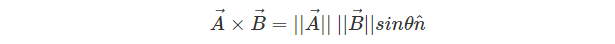• Call Now

1800-102-2727•

# Vector Products of Two Vectors

Vector Product of two vectors can be defined as the resultant vector perpendicular to both vectors. It is also known as the cross product of two vectors and is often denoted by a x b.

The Vector Product of two vectors results in a vector perpendicular to both vectors. The resultant vector can be obtained by applying the Right-Hand rule. In this article, we will discuss the properties of the vector product of two vectors and its several applications in detail.

Vector Product of two vectors is a form of binary multiplication of two vectors. Hence, if A and B are two vectors, then A x B denotes the resultant vector product of the two vectors, which is perpendicular to both A and B. Due to this, the Vector Product of two vectors is used to determine the perpendicular plane surface of both vectors in a three-dimensional space.

## Vector Product Formula

According to the definition, Vector Product for two vectors A and B is given by:
A × B = |A| |B| sin θ
where θ is the angle between the vectors

|A| |B| is the magnitude of vectors A and B
We can also represent the direction of the unit vector perpendicular to the plane containing the given two vectors using the formula.Here, ||A||, ||B|| are the magnitude of the given vectors
Θ is the angle between the corresponding vectors
n is the unit vector perpendicular to the plane containing A and B

## Properties of Vector Product

The following are the properties of the vector product of two vectors:-

1. The Vector Product is anticommutative. This denotes that if there exist two vectors, a and b, then the vector product of both the vector is anticommutative, i.e., a x b ≠ b x a.
2. The Vector Product is distributive. This denotes that if there exist two vectors, a and b, then the vector product of both the vector is distributive in nature i.e a x (b + c) = a x b + a x c
3. The Vector Product has zero vector property. This denotes that if there exist two vectors, a and b, then the vector product of both the vectors would be 0 only if a = 0 or b = 0.

## Vector Product of Different Vectors

Different cases of vectors result in different vector products. Some cases can include:-

1. Vector Product of Parallel Vector. We know that parallel vectors denote that the angle between the vectors is 0°. Let’s say there are two vectors X and Y with angle Θ between them, then if two vectors are parallel to each other, then the Vector Product isThus, the Vector Product of two vectors that are parallel to each other is a unit vector.

2. Vector Product of Perpendicular Vectors. We know that the angle between two vectors that are perpendicular to each other is 90°. Thus, if two vectors X and Y are said to be perpendicular to each other then the vector product formula gives the following resultant.Thus, the Vector Product of two vectors perpendicular to each other is equal to the dot product of their magnitudes. The Vector Product of two vectors that are perpendicular to each other is also known to represent the area of a rectangle.

## Points to Remember

1. To find the direction of the resultant vector obtained after the Vector Product of two vectors, you can apply the Right-hand rule.

You can follow the steps given below:-
• Align your index finger towards the direction of the first vector (A).
• Align the middle finger in the direction of the second vector. B
• Now the thumb points in the direction of the cross product of two vectors.
2. The Vector Product of unit vectors is 0. i x i = j x j = k x k = 0
3. The Vector Product of the vector itself is 0. a x a = 0.
4. The Vector Product with the Zero vector is 0. a x 0 = 0.
5. The Vector Product of the following are:-## Frequently asked questions on Vector Product of Two Vectors (FAQs)

1. Is a x b equivalent to b x a?
No, The magnitude of the Vector Product of a x b and b x a is the same. However, their direction differs; They are opposite to each other. Thus, effectively, a x b = - b x a.

2. Which rule is used to determine the direction of the Vector Product of two vectors?
The Right-Hand thumb rule is used to determine the direction of the plane perpendicular to the plane containing both vectors.Talk to our expert
Resend OTP Timer =
By submitting up, I agree to receive all the Whatsapp communication on my registered number and Aakash terms and conditions and privacy policy